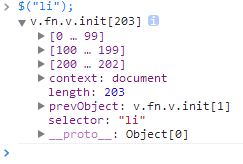# 从javascript判断一个对象是否为数组中学习js语法

1，真正的数组的判断方法

javascript中最简单的声明数组方法为：

var a = [];


a instanceof Array //true
a .constructor == Array //true


result = obj intanceof class


function Range(low, high) {
this.low = low;
this.high = high;
}
Range.prototype.constructor == Range; //true
Range.prototype = {
include: function(x){ return (x >= this.low && x <= this.high); },
exclude: function(x){ return (x < this.low && x > this.high); }
}
var r = new Range(0, 100);
r instanceof Range; //false
r instanceof Object; //true
Range.prototype.constructor == Objecct; //true


Range.prototype = {
include: function(x){ return (x >= this.low && x <= this.high); },
exclude: function(x){ return (x < this.low && x > this.high); }
}


Range.prototype = new Object({
include: function(x){ return (x >= this.low && x <= this.high); },
exclude: function(x){ return (x < this.low && x > this.high); }
});


The instanceof operator does not actually check whether r was initialized by the Range constructor. It checks whether it inherits from Range.prototype.

javascript中还有一个函数typeof具有与instanceof类似的功能，但是它返回的是具体的基本数据类型：number，string，function，object，undefined，boolean，只有这六种，不在这六种范围内的都返回object，也就是说typeof([])返回的是object，而不是array。

var a  = [];
a.constructor; //Array


2，伪数组的判断方法

javascript中有一种伪数组，它可以使用类似于Array的遍历方法进行遍历，有length属性获取元素的长度，可以使用[]下标来获取指定的元素，这种对象我们称之为伪数组，JQuery中的对象就是典型的伪数组，如下图：var is_array = function(value) {
return value &&
typeof value === 'object' &&
typeof value.length === 'number' &&
typeof value.splice === 'function' &&
!(value.propertyIsEnumerable('length'));
};